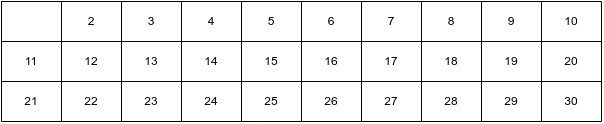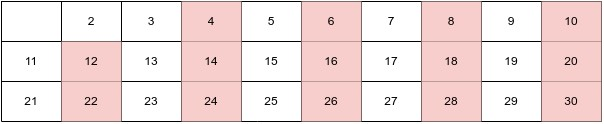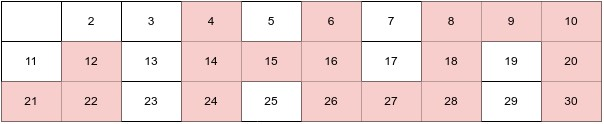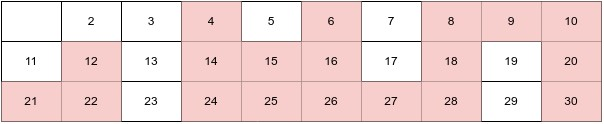×

Search anything:

# Complexity analysis of Sieve of Eratosthenes

#### Algorithms List of Mathematical AlgorithmsGet this book -> Problems on Array: For Interviews and Competitive Programming

In this article, we will discuss the Sieve of Eratosthenes method for identifying prime numbers, including its implementation, computational complexity, and alternative solutions.

We'll discuss:

• Introduction
• Brute Force Approach
• Sieve of Eratosthenes
• Example
• Implementation using Java
• Derive its Complexity
• Best, Average and Worst Case
• Conclusion

# Introduction

The Sieve of Eratosthenes is an ancient algorithm used for finding all prime numbers up to a given limit. It is named after the Greek mathematician Eratosthenes of Cyrene, who lived in the 3rd century BC. Eratosthenes was not only a mathematician, but also an astronomer, geographer, and poet. He is best known for his work on prime numbers and his algorithm for finding them, known as the Sieve of Eratosthenes.

# Brute Force Approach

In a brute force method, we iterate through a range of numbers, from 2 to `n`, and check the primality of each number. This process takes `√x` steps for each number `x` in the range, resulting in a complexity of `O(n √n)`. When `n` is large, this approach can be quite slow. To improve this, we can use an alternative algorithm known as the Sieve of Eratosthenes.

# Sieve of Eratosthenes

As the word ‘Sieve’ means filtering out dirt elements. The algorithm starts by creating a list of all integers from 2 to the given limit. The first step is to mark the number 2 as a prime number. Then, starting with 2, all multiples of that number are crossed off the list as they cannot be prime numbers. The next unmarked number, which is guaranteed to be a prime number, is 3, similarly all the multiple of 3 are crossed off. This process is continued for each subsequent unmarked number until all prime numbers up to the given limit have been identified.

The Sieve of Eratosthenes is a simple and efficient algorithm for finding prime numbers up to a given limit.

1. The algorithm is simple and easy to understand, making it a popular choice for teaching and learning about prime numbers.

2. The algorithm has a time complexity of `O(n log log n)` which makes it one of the most efficient algorithms for finding prime numbers.

3. It is a deterministic algorithm and does not rely on any randomness, so the results are always the same.

4. The algorithm can be easily implemented using a simple array or a more advanced data structure such as a bit array.

5. The algorithm has a wide range of applicability, it can be used to solve many number-theoretic problems, such as finding the largest prime factor of a given number, or counting the number of prime numbers within a given range.

1. The algorithm has a space complexity of `O(n)` which can be quite high when the upper limit `n` is large, this can be a problem for memory-constrained systems.

2. The algorithm is not suitable for concurrent or parallel execution, which makes it less efficient on modern multi-core processors.

3. The algorithm is not as efficient as other algorithms for finding prime numbers when the upper limit `n` is large.

4. It's not suitable for testing very large prime numbers because it's not practical, as it will require too much memory and time.

# Example

Here is an example of how the algorithm works for n = 30:

1. Create a list of numbers from 2 to 30 and mark them all as prime.1. Starting with `2`, cross out every multiple of 2 in the list. The remaining numbers are: 2, 3, 5, 7, 9, 11, 13, 15, 17, 19, 21, 23, 25, 27, 29.1. Move to the next number in the list `3` and cross out every multiple of `3`. The remaining numbers are: 2, 3, 5, 7, 11, 13, 17, 19, 23, 25, 29.1. Repeat this process with the next number in the list `5` and cross out every multiple of `5`. The remaining numbers are: 2, 3, 5, 7, 11, 13, 17, 19, 23, 29.1. Continue this process until you reach the square root of `30`. The remaining numbers in the list are all prime numbers up to `30`: 2, 3, 5, 7, 11, 13, 17, 19, 23, 29.So, the prime numbers from 1 to 30 are 2, 3, 5, 7, 11, 13, 17, 19, 23, 29.

# Implementation using Java

Here is an example of Sieve of Eratosthenes using Java language:

``````import java.util.Arrays;

public class SieveOfEratosthenes {
public static void main(String[] args) {
int n = 30; // upper limit for finding prime numbers
boolean[] primes = sieve(n);
for (int i = 2; i <= n; i++) {
if (primes[i]) {
System.out.print(i + " ");
}
}
}

public static boolean[] sieve(int n) {
boolean[] primes = new boolean[n + 1];
Arrays.fill(primes, true); // Initially assume all numbers are prime
primes = primes = false; // 0 and 1 are not prime numbers
for (int i = 2; i * i <= n; i++) {
if (primes[i]) {
// Mark all multiples of i as not prime
for (int j = i * i; j <= n; j += i) {
primes[j] = false;
}
}
}
return primes;
}
}
``````

In the main method, it first declares an integer variable `n` and assigns it the value of 30, which represents the upper limit for finding prime numbers. Then it calls the `sieve` method and assigns the returned boolean array to the variable `primes`. After that, it iterates through the boolean array, starting from 2, and for each element in the array, it checks if it's marked as true, if it is, it prints the number.

The `sieve` method takes an integer `n` as an input, which is the upper limit for finding prime numbers. It creates a boolean array `primes` of size `n+1`, initially sets all numbers as prime using `Arrays.fill(primes, true)` method. Then it sets 0 and 1 to be not prime numbers using `primes = primes = false`.

It then iterates through the array starting from 2, and for each element, it checks if it's marked as prime. If it is, it marks all multiples of that number as composite, by iterating through the array again, starting from `i*i` (to avoid marking multiples that have already been marked as composite) and ending at `n`. The inner loop increments the index by `i`, so it will mark all multiples of `i` as not prime.

# Derive its Complexity

The outer loop starts at 2 and runs until i * i <= n, which means that it runs in O(√n) time.

The inner loop starts at i*i and runs until j is less than or equal to n, marking all multiples of i as not prime. The number of iterations of the inner loop is n/i.

The inner loop is executed for each value of i in the outer loop, and since i is increasing, the number of iterations of the inner loop decreases. Therefore, the total number of iterations of the inner loop is the sum of the number of iterations for each value of i in the outer loop, which can be represented mathematically as:

Σ(n/i), where 2 <= i <= √n

So, Σ(n/i) = n/2 + n/3 + n/4 + n/5 +.......+ n/√n
= n(1/2 + 1/3 + 1/4 + 1/5 +.......+ 1/√n)

and (1/2 + 1/3 + 1/4 +.......+ 1/√n) ≈ log(log n)

Hence, Σ(n/i) = n(log(log n))

By using the property of prime number distribution, we can approximate this sum as n log log n. Therefore, the overall time complexity of this code is `O(n log(log n)).`

The space complexity of the algorithm is `O(n)` as we are using a boolean array of size n+1 to store the prime numbers.

# Best, Average and Worst Case

Best Case: When the value of n is 2, the outer loop in the sieve() method will iterate only once, from 2 to √2 = 1, and the inner loop will not execute at all. Therefore the time complexity for this case is `O(1)` which is constant time complexity.

Average case: The outer loop will iterate from 2 to √n and the inner loop will iterate from i^2 to n, marking all multiples of i as non-prime. The number of iterations of the inner loop is n/i. The total number of iterations is the sum of the number of iterations for all prime numbers, which is n/2 + n/3 + n/4 + ... + n/√n.Therefore the time complexity for this case is `O(n log(log n))`.

Worst case: The time complexity of the Sieve of Eratosthenes algorithm in worst case is also `O(n log(log n))` where n (a large number) is the range of numbers to find primes. The reason is the same as discussed in the average case.

It's worth mentioning that the sieve of Eratosthenes algorithm has a time complexity of `O(n log(log n))` which is considered efficient for finding prime numbers.

Cases Time Complexity
Best Case O(1)
Average Case O(n log(log n))
Worst Case O(n log(log n))

# Conclusion

In conclusion, the Sieve of Eratosthenes is a simple and efficient algorithm for finding prime numbers up to a given limit. It has a time complexity of `O(n log(log n))` and it's easy to understand and implement. However, it has a space complexity of `O(n)` which can be a problem for memory-constrained systems. The Sieve of Eratosthenes remains a popular choice for finding prime numbers due to its simplicity and wide range of applicability.#### Abhijeet Saroha

Abhijeet Saroha is a Machine Learning Developer, Intern at OpenGenus and is pursuing Bachelor of Technology in Information Technology at Delhi University.